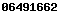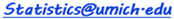## Binomial Distribution

 n p a b P(a<=X<=b), where X=#successes(prob=p) in N trials

## Normal Distribution

 z % Area on [-z : z]

## Student 's T Distribution

 t n (d.f.) % Area on [-t : t]

## Chi Square Distribution

 ChiSq n  (d.f.) p (right tail)

## Fisher's F Distribution

 F n1 (df1) n2 (df2) p (right tail)
SOCR Resource Visitor number